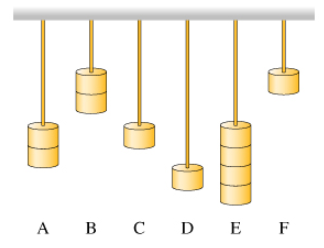# Problem: A combination work of art/musical instrument is illustrated. Six pieces of identical piano wire (cut to different lengths) are hung from the same support, and masses are hung from the free end of each wire. Each wire is 1, 2, or 3 units long, and each supports 1, 2, or 4 units of mass. The mass of each wire is negligible compared to the total mass hanging from it. When a strong breeze blows, the wires vibrate and create an eerie sound.(a) Rank each wire-mass system on the basis of its fundamental wavelength.(b) Rank each system on the basis of its wave speed.(c) Rank each system on the basis of its frequency.

###### FREE Expert Solution

Part A:

Wavelength:

$\overline{){\mathbf{\lambda }}{\mathbf{=}}{\mathbf{2}}{\mathbit{L}}}$

79% (302 ratings)###### Problem Details

A combination work of art/musical instrument is illustrated. Six pieces of identical piano wire (cut to different lengths) are hung from the same support, and masses are hung from the free end of each wire. Each wire is 1, 2, or 3 units long, and each supports 1, 2, or 4 units of mass. The mass of each wire is negligible compared to the total mass hanging from it. When a strong breeze blows, the wires vibrate and create an eerie sound.

(a) Rank each wire-mass system on the basis of its fundamental wavelength.
(b) Rank each system on the basis of its wave speed.
(c) Rank each system on the basis of its frequency.